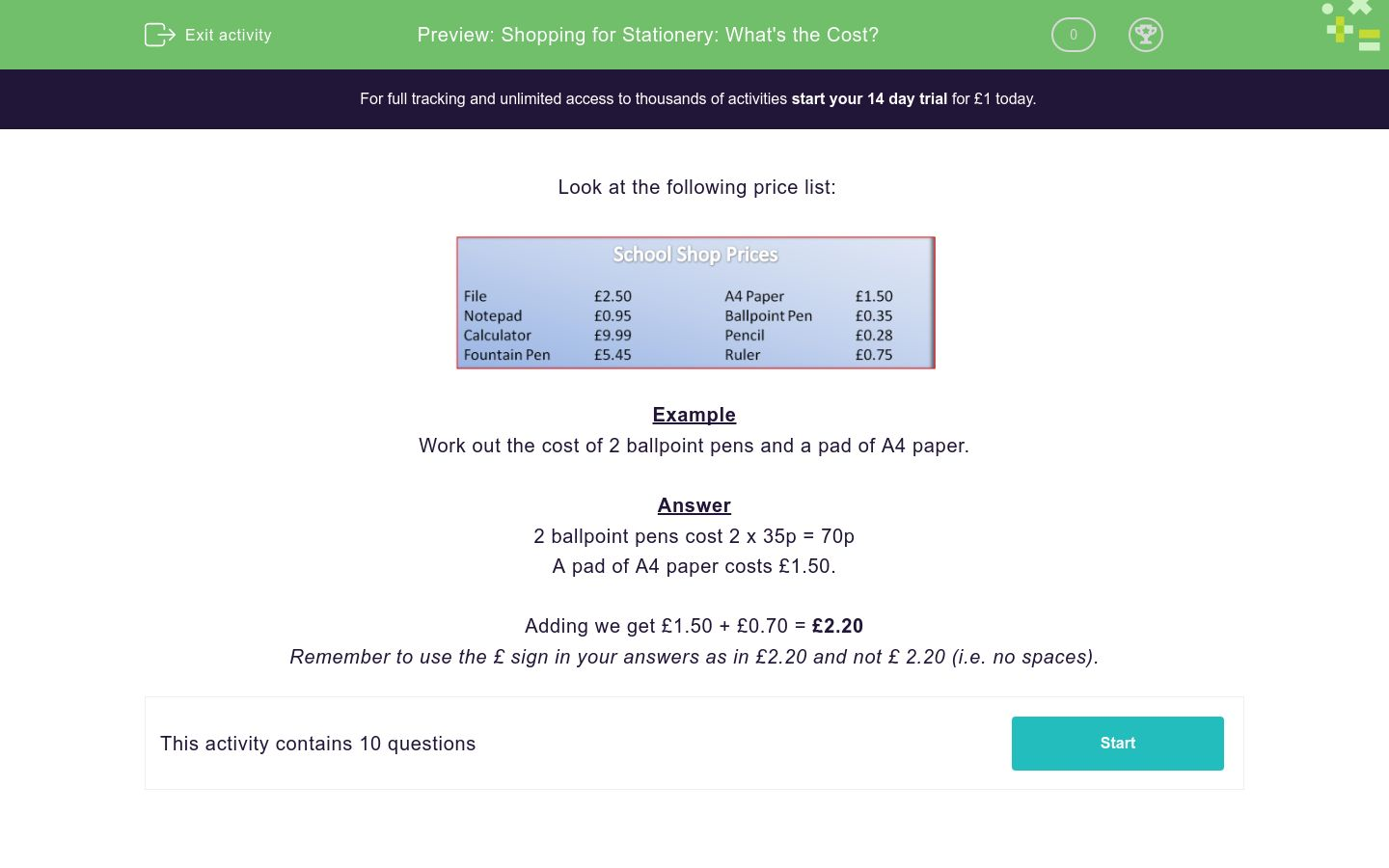# Shopping for Stationery: What's the Cost?

In this worksheet, students work out the cost of items they might need for school.Key stage:  KS 2

Curriculum topic:   Measurement

Curriculum subtopic:   Solve Four Operations Problems

Difficulty level:### QUESTION 1 of 10

Look at the following price list:Example

Work out the cost of 2 ballpoint pens and a pad of A4 paper.

2 ballpoint pens cost 2 x 35p = 70p

A pad of A4 paper costs £1.50.

Adding we get £1.50 + £0.70 = £2.20

Look at the following price list:How much does Jack spend when he buys 3 pencils?  Answer in £s.

Look at the following price list:How much does Jill spend when she buys 2 pencils and a ruler?  Answer in £s.

Look at the following price list:How many files can be bought with a £10 note?

Look at the following price list:How much change will I get from a £10 note if I buy a fountain pen and a ballpoint pen?

Look at the following price list:What is the total cost of five calculators?

Look at the following price list:What is the total cost of one of each item on sale at the school shop?

Look at the following price list:How many of the cheapest item could be bought with a £5 note?

Look at the following price list:How much more expensive is a calculator compared to a fountain pen?

Look at the following price list:How much will 3 notepads and 4 ballpoint pens cost?

Look at the following price list:The shopkeeper decides to sell the A4 paper at half price.

How many pads can now be bought for £5?

• Question 1

Look at the following price list:How much does Jack spend when he buys 3 pencils?  Answer in £s.

£0.84
EDDIE SAYS
Pencils cost 28p 28 x 3 = £0.84
• Question 2

Look at the following price list:How much does Jill spend when she buys 2 pencils and a ruler?  Answer in £s.

£1.31
EDDIE SAYS
Pencils are 28p and a ruler is 75p 28 x 2 pencils = 56p £0.56 + £0.75 = £1.31
• Question 3

Look at the following price list:How many files can be bought with a £10 note?

4
EDDIE SAYS
Files are £2.50. 250 x 4 = 10 4 files can be bought for ten pounds.
• Question 4

Look at the following price list:How much change will I get from a £10 note if I buy a fountain pen and a ballpoint pen?

£4.20
EDDIE SAYS
£5.45 + £0.35 = £5.80 £10.00 - £5.80 = £4.20
• Question 5

Look at the following price list:What is the total cost of five calculators?

£49.95
EDDIE SAYS
£9.99 x 5 = £49.95
• Question 6

Look at the following price list:What is the total cost of one of each item on sale at the school shop?

£21.77
EDDIE SAYS
2.50 + 0.95 + 9.99 + 5.45 + 1.50 + 0.35 + 0.28 + 0.75 = £21.77
• Question 7

Look at the following price list:How many of the cheapest item could be bought with a £5 note?

17
EDDIE SAYS
.28 x 17 = 4.76 17 pencils can be bought with a 5 pound note.
• Question 8

Look at the following price list:How much more expensive is a calculator compared to a fountain pen?

£4.54
EDDIE SAYS
9.99 - 5.45 = 4.54
• Question 9

Look at the following price list:How much will 3 notepads and 4 ballpoint pens cost?

£4.25
EDDIE SAYS
Notepads: 0.95 x 3 = 2.85 Ballpoint pens: 0.35 x 4 = 1.40 2.85 + 1.40 = 4.25
• Question 10

Look at the following price list:The shopkeeper decides to sell the A4 paper at half price.

How many pads can now be bought for £5?

6
EDDIE SAYS
1.50 divided by 2 = 0.75 is half price 0.75 x 6 = 4.50 6 packs of A4 paper can be bought for 5 pounds.
---- OR ----

Sign up for a £1 trial so you can track and measure your child's progress on this activity.

### What is EdPlace?

We're your National Curriculum aligned online education content provider helping each child succeed in English, maths and science from year 1 to GCSE. With an EdPlace account you’ll be able to track and measure progress, helping each child achieve their best. We build confidence and attainment by personalising each child’s learning at a level that suits them.

Get started